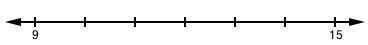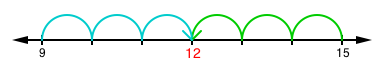### Home > GC > Chapter 7 > Lesson 7.2.6 > Problem7-95

7-95.

For each pair of numbers, find the number that is exactly halfway between them.

1. $9$ and $15$

It may help to draw a number line.Count towards the middle from both sides.1. $3$ and $27$

Find the average of the two numbers.

$\frac{3+27}{2}= \frac{30}{2}=15$

1. $10$ and $21$